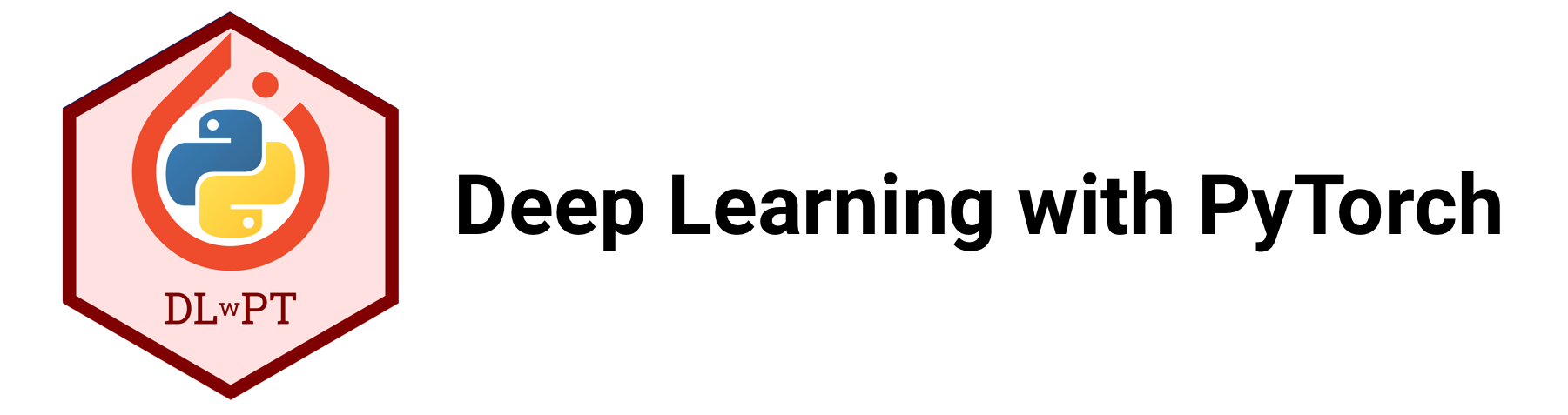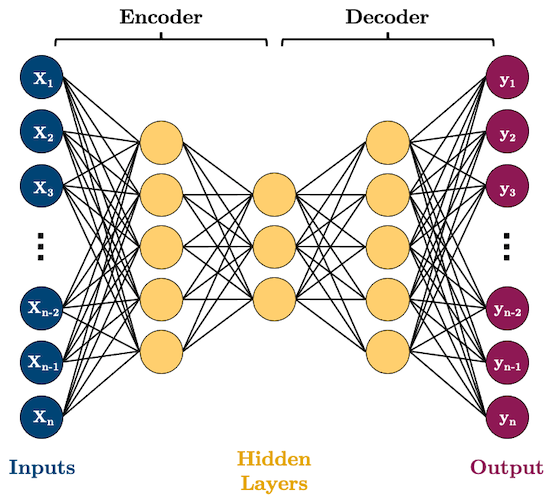# Chapter 7: Advanced Deep Learning¶

By Tomas Beuzen 🚀## Chapter Learning Objectives¶

• Describe what an autoencoder is at a high level and what they can be useful for.

• Describe what a generative adversarial network is at a high level and what they can be useful for.

• Describe what a multi-input model is and what they can be useful for.

## Imports¶

import numpy as np
import pandas as pd
import torch
from torch import nn, optim
from torch.utils.data import DataLoader, TensorDataset
from torchvision import transforms, datasets, utils, models
from torchsummary import summary
from sklearn.datasets import make_blobs
from sklearn.preprocessing import StandardScaler
from utils.plotting import *
import matplotlib.pyplot as plt
import matplotlib.animation as animation
from IPython.display import HTML
from PIL import Image
plt.style.use('ggplot')
plt.rcParams.update({'font.size': 16, 'axes.labelweight': 'bold', 'axes.grid': False})


## 1. Autoencoders¶

Autoencoders (AE) are networks that are designed to reproduce their input at the output layer. They are composed of an “encoder” and “decoder”. The hidden layers of the AE are typically smaller than the input layers, such that the dimensionality of the data is reduced as it is passed through the encoder, and then expanded again in the decoder:Why would you want to use such a model? As you can see, AEs perform dimensionality reduction by learning to represent your input features using fewer dimensions. That can be useful for a range of tasks but we’ll look at some specific examples below.

### 1.1. Example 1: Dimensionality Reduction¶

Here’s some synthetic data of three features and two classes. Can we reduce the dimensionality of this data to two features while preserving the class separation?

n_samples = 500
X, y = make_blobs(n_samples, n_features=2, centers=2, cluster_std=1, random_state=123)
X = np.concatenate((X, np.random.random((n_samples, 1))), axis=1)
X = StandardScaler().fit_transform(X)
plot_scatter3D(X, y)